# Surveying - 1

## 10 Questions MCQ Test Mock Test Series for Civil Engineering (CE) GATE | Surveying - 1

Description
This mock test of Surveying - 1 for GATE helps you for every GATE entrance exam. This contains 10 Multiple Choice Questions for GATE Surveying - 1 (mcq) to study with solutions a complete question bank. The solved questions answers in this Surveying - 1 quiz give you a good mix of easy questions and tough questions. GATE students definitely take this Surveying - 1 exercise for a better result in the exam. You can find other Surveying - 1 extra questions, long questions & short questions for GATE on EduRev as well by searching above.
QUESTION: 1

### The sensitiveness of a bubble tube in a level would decrease if

Solution:

Sensitivity of a bubble tube/ level tubs is its capability of exhibiting small deviation of the tube horizontally.

Sensitivity increases when:
Temperature Decreases
Larger diameter
Larger Length
Higher length of one division
Higher smoothness of finish of internal surface of liquid
Low surface tension and viscosity

QUESTION: 2

### Hydrographic surveys deal with the mapping of

Solution:

Types of survey on the basis of place
Land Survey: Done on Land
Hydrographic Survey: Survey of water bodies like streams, sea, ponds etc.
Underground Survey: required for construction of tunnels.
Aerial Survey: Aerial photographs via aeroplanes, drones etc. Preparation of large scale maps of an area.

QUESTION: 3

### If "L" is the length of the chain , "W" is the weight of the chain and 'T' is the tension , the Sag Correction for the chain line is

Solution:

When tape is supported at two ends then it will always sag downwards due to its own weight, thus, apparent length larger than the given length. The correction is always negative and is given by-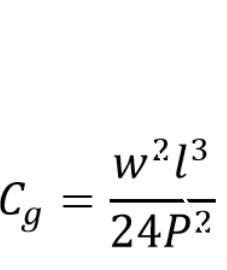C_g=(w2 l3)/(24P2 )
w weight per unit length
P applied pull
l  length of tape suspended between the supports.

QUESTION: 4

If the whole circle bearing is 315°20′, its quadrantal bearing would be

Solution:

As it can be seen from the figure the quadrantal bearing is N44°40′W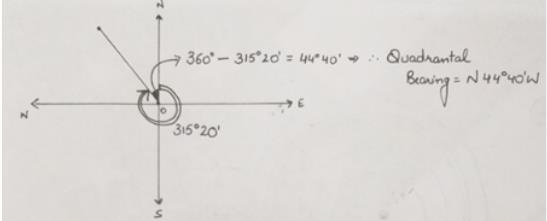*Answer can only contain numeric values
QUESTION: 5

The radius of a 1 degree curve is __________ m

Solution: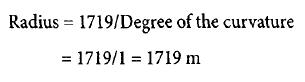QUESTION: 6

A level when set up 25 m from peg A and 50 m from peg B reads 2.847 on a staff held on A and 3.462 on a staff held on B, keeping bubble at its centre while reading. If the reduced levels of A and B are 283.665 m and 284.295 m respectively, the collimation error per 100 m is

Solution:

Let the collimation error per 100m is e
Correction for staff reading at A = (25/100)e= 0.25e
Correction for staff reading at B = (50/100)e =0.5e
True staff reading at A = Observed Value + Correction = 2.847+0.25e
True staff reading at B = 3.462+0.5e
Difference in elevation = RLa – RLb =283.665-284.295= -0.63m
=2.847+0.25e-(3.462+0.5e) = -0.615-0.25e =-0.63

e = 0.06m

QUESTION: 7

An invar tape, 50 m in length, standardized at 20oC temperature and 10kg pull, is used to measure a baseline. The Correction per tape length, if at the time of measurement the temperature was 30o and the coefficient of linear expansion of the tape was 1x10-6 per oC , will be

Solution:

Correction per tape length is given by: (Coefficient of Linear Expansion) x (Change in Temperature) x (Tape Length)
= 10-6 x (30-20) x 50 = 0.0005 m

QUESTION: 8

A Rectangular plot of 16km2  in area is shown on a map by a similar rectangular area of 1 cm2 , R.F of the scale to measure a distance of 40km will be

Solution:

Representative fraction of the map (in terms of length) =√((Area on Map)/(Actual Area of plot))
Area on Map = 1 cm2 = 1 x 10-6 m2
Actual Area of plot = 16 km2 = 16 x 106 m2
Representative fraction of the map (in terms of length) = √(〖10〗(-6)/(16×〖10〗6 )) =1/4000000

QUESTION: 9

Following observations were taken with a transit fitted with stadia wires.
The line of sight was horizontal and the staff was held vertical.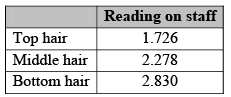The tacheometric constants k and C are 100 and 0.4 m, respectively. The horizontal distance between staff and instrument is

Solution:

D = KS + C

S  = 2.830 - 1.726 = 1.104

D = 100 x 1.104 + 0.4 = 110.8m

QUESTION: 10

Following observations were taken during a reciprocal leveling: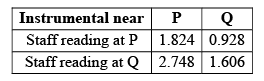If reduced level of P is 140.815 m, the reduced level of Q is

Solution: